Previous in Forum: Water Supply Pipe in Utility Trenches Next in Forum: Waste water treatment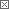Anonymous Poster

#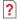Please help an engineering-student.

11/27/2008 4:03 PM

Hello

I have added a link to a picture with a problem here. Let me just first say that I do not need help with the calculations and this is not a homework problem.

I have done the calculations and gotten the right answers, but the problem is that I really don't understand what is happening.

It is question c that is the problem:

http://img217.imageshack.us/my.php?image=utennavnxf7.jpg

The way I obtained the right answer was to put the force in the rod at B=0 and the force at A=P (moment-equlibrium). The I said that the displacement at A caused by the stress in the rod and the temperature increase was twice the displacement at B because of the temperature increase(remember, no force here since it is slacking). And the displacement at A is twice as much as at B because the triangle is rigid.

But why does this actually work? I mean, how do I understand that at some point when the temperature rises to a certain point, the force in rod B will be 0? Could someone please help me understand this.

Interested in this topic? By joining CR4 you can "subscribe" to

### Comments rated to be Good Answers: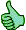Check out these comments that don't yet have enough votes to be "official" good answers and, if you agree with them, rate them!
Anonymous Poster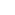#1

###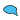Re: Please help an engineering-student.

11/27/2008 4:55 PM

Because the triangle arm A-C is rigid, both rods will grow the same length - but the arc length of the path of the triangle is different, at some point the rod B will be longer than the distance travelled by point B.

I'm not sure I have clearly explained it -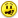; I can easily picture the answer in my mind I am just finding a hard time putting it to words - it would be a lot easier if I could sketch it in person to show you.

Just think about the arc lengths of point B and A.2
Guru

Join Date: Nov 2007
Posts: 1212#2

###Re: Please help an engineering-student.

11/27/2008 6:33 PM

If you have done parts (a) and (b), you should have no difficulty understanding what is happening in part (c).

(a) From equilibrium, TA(2b) + TB(b) = P(2b)

Also, TB = TA/2 because the strain at B is only half of that at A.

Solving the above two equations, we get TA = 0.8P and TB = 0.4P

If P is 500#, then TA = 400# and TB = 200# which is the answer to part (a)

(b) I leave it to you.

(c) When wire B goes slack, wire A carries all of the force necessary to restore equilibrium, so at some temperature X, TA = P and TB = 0.

ΔA = 500*L/120000 + X*L*12.5E-6

ΔB = X*L*12.5E-6 = ΔA/2

Solving, X = 333oF, or a further increase of 153oF from 180oF.

__________________
Bruce
Power-User

Join Date: Jan 2008
Location: since 20 Jan 09, the USSA
Posts: 375#3

###Re: Please help an engineering-student.

11/27/2008 11:35 PM

Point A moves farther for a given weight at point D than does Point B, because A is farther from the pivot point. If the two wires undergo equal elongation due to temperature rise, then at some point the wire at B will be longer than the distance between B and the tether point for the wire for point B. At this point, the entire weight hanging off point D will be carried by the tether to point A, and the tether to point B will have no stress on it whatsoever.

So if this were a real problem, and you had to take into account temperature effects, you would have to look at different wire materials so that the thermal coefficients of expansion were in such a ratio that both tethers continued to do their jobs, or you would have to oversize the tether at point A so that it could do the job by itself. Or maybe not all by itself, but with diminished support at point B.

This is almost like designing a bimetallic strip to perform a particular function at a particular electrical current or temperature.

Power-User

Join Date: Mar 2008
Location: Globaly - very close to the southern most point of Canada
Posts: 445#4

###Re: Please help an engineering-student.

11/28/2008 10:29 AM

If you want to calculate it to understand it, give the opposite ends of the wires a point. Say the opposite end of A is E and the opposite end of B is F.

Trig triangles C-B-F and C-A-E and compare angle C in each case. (assuming the wire is straight in each case)

You should note one angle in a triangle is less. When that angle applied to the other triangle, the wire is too long and therefore in practice will sag or bow.

__________________
-why bother doing it wrong when it will be anyway.......
Guru

Join Date: Nov 2007
Posts: 1212#5

###Re: Please help an engineering-student.

11/28/2008 12:25 PM

Point B is constrained to move exactly one half of the distance that Point A moves, no matter what the temperature is because the triangle rotates about Point C as a rigid body.

When there is no temperature effect, the force in Wire B will be only half of the force in Wire A because stress is proportional to strain (Hooke's Law).

When the temperature starts to rise, both wires elongate equally. As this occurs, the tension in Wire B decreases and the tension in Wire A increases. If the temperature is elevated to the point where thermal expansion of Wire A is equal to its tensile strain, then Point A deflects twice as much as the thermal expansion of Wire B, so Wire B requires no force to hold it where it is constrained to be.

If the temperature is elevated further, Wire A expands and Wire B sags.

__________________
Bruce
Interested in this topic? By joining CR4 you can "subscribe" to